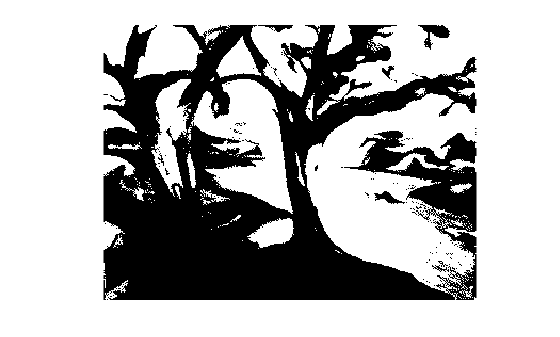im2bw

Convert image to binary image, based on threshold

Description

example

BW = im2bw(I,level) converts the grayscale image I to binary image BW, by replacing all pixels in the input image with luminance greater than level with the value 1 (white) and replacing all other pixels with the value 0 (black).

This range is relative to the signal levels possible for the image's class. Therefore, a level value of 0.5 corresponds to an intensity value halfway between the minimum and maximum value of the class.

BW = im2bw(X,cmap,level) converts the indexed image X with colormap cmap to a binary image.

BW = im2bw(RGB,level) converts the truecolor image RGB to a binary image.

Examples

collapse all

BW = im2bw(X,map,0.4);
imshow(X,map), figure, imshow(BW)Input Arguments

collapse all

2-D grayscale image, specified as an m-by-n numeric matrix.

Data Types: single | double | int16 | uint8 | uint16

2-D indexed image, specified as an m-by-n numeric matrix.

Data Types: single | double | int16 | uint8 | uint16

Colormap associated with indexed image X, specified as a c-by-3 numeric matrix with values in the range [0, 1]. Each row is a three-element RGB triplet that specifies the red, green, and blue components of a single color of the colormap.

Data Types: single | double | int16 | uint8 | uint16

2-D RGB image, specified as an m-by-n-by-3 numeric matrix.

Data Types: single | double | int16 | uint8 | uint16

Luminance threshold, specified as a number in the range [0, 1]. To compute level, you can use the graythresh function.

Data Types: single | double | int16 | uint8 | uint16

Output Arguments

collapse all

Binary image, returned as an m-by-n logical matrix.

Data Types: logical

Algorithms

If the input image is not a grayscale image, im2bw converts the input image to grayscale using ind2gray or rgb2gray, and then converts this grayscale image to binary by thresholding.

Compatibility Considerations

expand all

Not recommended starting in R2016a